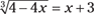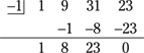176.    x = –1

First, add 3 to each side of the equation.Now raise each side of the equation to the third power.

4 – 4x = x 3 + 9x 2 + 27x + 27

Set the equation equal to 0 and solve for x.

0 = x 3 + 9x 2 + 31x + 23

You can use synthetic division to find a solution/factor; try x = –1.x = –1 is a solution, so x + 1 is a factor of the polynomial. You can write x 3 + 9x 2 + 31x + 23 as (x + 1)(x 2 + 8x + 23), using the coefficients in the bottom row of the synthetic division.

The quadratic doesn’t factor, and, using the quadratic formula, you get imaginary

numbers. The imaginary/complex answers are.

So the only possible real answer is x = –1.

Check x = –1 using the original equation.So the solution x = –1 works.

177.    x = 13

Square both sides of the equation.Now add –5 to both sides.Square both sides again.

x + 3 = 16

Solve for x.

x = 13

Check x = 13 in the ...

Get 1001 Algebra II Practice Problems For Dummies now with the O’Reilly learning platform.

O’Reilly members experience books, live events, courses curated by job role, and more from O’Reilly and nearly 200 top publishers.